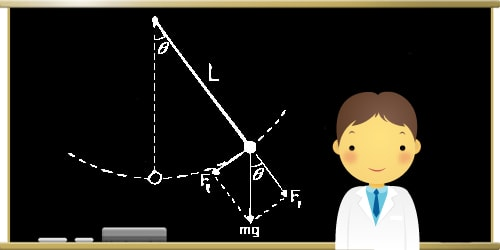# “Pendulum Waves” …. What is a pendulum?~~July 7, 2014~~

pendulum is a weight suspended from a pivot so that it can swing freely. When a pendulum is displaced sideways from its resting equilibrium position, it is subject to a restoring force due to gravity that will accelerate it back toward the equilibrium position. When released, the restoring force combined with the pendulum’s mass causes it to oscillate about the equilibrium position, swinging back and forth.The time for one complete cycle, a left swing and a right swing, is called the period. The period depends on the length of the pendulum, and also to a slight degree on the amplitude, the width of the pendulum’s swing.

From its discovery in around 1602 by Galileo Galilei, the regular motion of pendulums was used for timekeeping, and was the world’s most accurate timekeeping technology until the 1930s. Pendulums are used to regulate pendulum clocks, and are used in scientific instruments such as accelerometers and seismometers. Historically they were used as gravimeters to measure the acceleration of gravity in geophysical surveys, and even as a standard of length. The word ‘pendulum’ is new Latin, from the Latin pendulus, meaning ‘hanging’.

The simple gravity pendulum is an idealized mathematical model of a pendulum. This is a weight (or bob) on the end of a massless cord suspended from a pivot, without friction. When given an initial push, it will swing back and forth at a constant amplitude. Real pendulums are subject to friction and air drag, so the amplitude of their swings declines.~~SOURCE~~

http://en.wikipedia.org/wiki/Pendulum# ~~Pendulum Waves~~

Harvard Natural Sciences Lecture Demonstrations